• 目前网络电子地图的使用很普遍。利用电子地图可以很方便地确定从一个地点到另一个地点的路径。特别地，可确定在城市中的公交...你的任务：对给定的一个（无向）图G，及G中的两点s、t，计算从起点s到顶点t的最短距离
• 我试图用Dijkstra算法在真实地图上找到两个点之间的最短路径，但是在有限的时间内(120秒)我无法得到结果。如何优化我的代码？我只允许用“枕头”作为外包装。在在map上有两个红点(33193)(749457)，我想画出它们中...
我试图用Dijkstra算法在真实地图上找到两个点之间的最短路径，但是在有限的时间内(120秒)我无法得到结果。如何优化我的代码？我只允许用“枕头”作为外包装。在在map上有两个红点(33193)(749457)，我想画出它们中最短的路径。但即使花费很长时间也不能得出结果。我很好地测试了Dijkstra的算法，不知道它是否还有很多缺陷。Dijkstra的算法是用同样的距离创建的——1。在Here是完整的代码。在def walkback(P, x, y):L = [y]while x != y:y = P[y]L = [y] + Lreturn Ldef path(A, x, y):M = []W = [x]P = {}while W != []:u = W.pop()if u == y:return walkback(P, x, y)M.append(u)for v in A[u]:if not v in M:P[v] = uW.append(v)return None这是Dijkstra算法。我为此做了测试。在^{pr2}\$对于任何像素，白色和红色(开始、结束)都是可移动的点，并设置为0。其他颜色设置为1。在for x in range(0, w):for y in range(0, h):(r, g, b, a) = im.getpixel((x, y))if (r, g, b) == (255, 0, 0):Red.append((x, y))arr[y][x] = 0elif (r, g, b) == (255, 255, 255):arr[y][x] = 0else:arr[y][x] = 1这就为任何可移动的点创建了一个图形。对于['0.1'：['0.2'，'0.3']]，这意味着您可以从(0，1)移动到(0，2)和(0，3)。在def coord2num(x, y):z = str(x) + "." + str(y)return zB = {}for x in range(0, h):for y in range(0, w):if arr[x][y] != 0:continuekey = coord2num(x, y)B[key] = []if x - 1 >= 0 and arr[x - 1][y] == 0:value = coord2num(x - 1, y)B[key].append(value)if x + 1 < h and arr[x + 1][y] == 0:value = coord2num(x + 1, y)B[key].append(value)if y + 1 < w and arr[x][y + 1] == 0:value = coord2num(x, y + 1)B[key].append(value)if y - 1 >= 0 and arr[x][y - 1] == 0:value = coord2num(x, y - 1)B[key].append(value)我期望输出最短的路径，但它仍然没有时间。在
展开全文• 地图上显示有多个分散的城市和一些连接城市的快速道路。 每条道路都有着距离和人流量 二个指标。人流量越大越容易有堵塞的意外发生。 每次救援的时候，都会给你起点和终点。 要求你输出到达终点的最短距离和对应的...
最短距离和人流量
题目：

作为一个城市(城市标号从1开始)的应急救援队伍的负责人，你有一张特殊的全国地图。在地图上显示有多个分散的城市和一些连接城市的快速道路。
每条道路都有着距离和人流量 二个指标。人流量越大越容易有堵塞的意外发生。
每次救援的时候，都会给你起点和终点。 要求你输出到达终点的最短距离和对应的人流量和（如果最短距离有多条路线，人流量总和小的优先选择）
输入
输入n,m ， n个城市m条道路。 然后m行个 a b c d
表示 a 和b 之间有一条双向边，长度为 c  ，人流量为d
最后一行是 s ，e
s为救援起点 ，e为终点
n<1000
输出
输出 一行 有2个数， 最短距离和 人流量总和
样例输入
3 2
1 2 5 6
2 3 4 5
1 3
0 0
样例输出
9 11
不知道Dijkstra 还是先去学一下吧

代码：
#include <stdio.h>
#inc lude <string.h>
#include <algorithm>
#include<iostream>
using namespace std;
const int inf = 8888888;
int n,m;
int map_len,map_time;
int vis,cost_len,cost_time;

void Dijkstra(int s,int e)
{
int i,j,min,pos;
memset(vis,0,sizeof(vis));
cost_len[s] = 0;
cost_time[s] = 0;
vis[s] = 1;
for(i = 0; i<n; i++)
{
cost_len[i] = map_len[s][i];
cost_time[i] = map_time[s][i];
}
for(i = 1; i<n; i++)
{
min = inf;
for(j = 0; j<n; j++)
{
if(cost_len[j]<min && !vis[j])
{
pos = j;
min = cost_len[j];
}
}
vis[pos] = 1;
for(j = 0; j<n; j++)
{
if(cost_len[pos]+map_len[pos][j]<cost_len[j] && !vis[j])
{
cost_len[j] = cost_len[pos]+map_len[pos][j];
cost_time[j] = cost_time[pos]+map_time[pos][j];
}
else if(cost_len[pos]+map_len[pos][j]==cost_len[j] && !vis[j])//路程相同的情况下找时间少的
{
if(cost_time[pos]+map_time[pos][j]<cost_time[j])
{
cost_time[j] = cost_time[pos]+map_time[pos][j];
}
}
}
}
}

int main()
{
int s,e,len,time;
int i,j;
scanf("%d%d",&n,&m);

for(i = 0; i<100; i++)
{
for(j = 0; j<100; j++)
map_len[i][j] = map_time[i][j] = inf;
map_len[i][i] = map_time[i][i] = 0;
}
while(m--)
{
scanf("%d%d%d%d",&s,&e,&len,&time);
s--;
e--;
if(len<map_len[s][e])
{
map_len[s][e] = map_len[e][s] = len;
map_time[s][e] = map_time[e][s] = time;
}
}

scanf("%d%d",&s,&e);
s--;
e--;
Dijkstra(s,e);
printf("%d %d\n",cost_len[e],cost_time[e]);

return 0;
}


编译样例：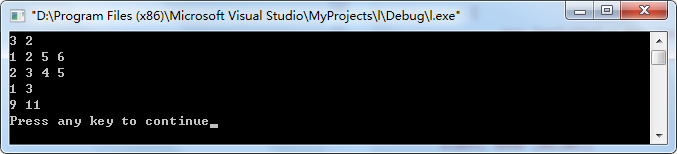展开全文• 为何地图上的航线是曲线如果我们观察地图上的航线，就会发现航线是弯曲的。基本上可以认为地球是个球体，如果飞机在两个城市之间飞行，最好的飞行线路是取这两个城市之间的最短距离。这其实课看成球面上任意两点之间...
为何地图上的航线是曲线如果我们观察地图上的航线，就会发现航线是弯曲的。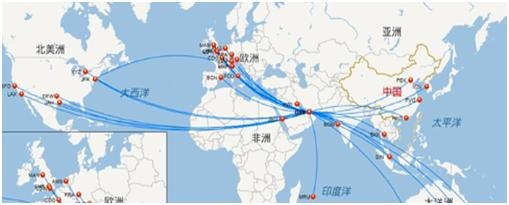基本上可以认为地球是个球体，如果飞机在两个城市之间飞行，最好的飞行线路是取这两个城市之间的最短距离。这其实课看成球面上任意两点之间的最短距离。过球面上的任意两点以及球心可以做一个截平面，与球面的截痕为一个圆，这个圆的大小不随两点不同而变化，半径都是球半径。这个圆是任意平面与球面相截得到的所有不同的圆中，半径最大的，因此叫做大圆。而只要你沿着球表面做线连接任意两个点，曲线长度最短的一定是这个大圆的劣弧长度。航线按两个城市之间的大圆弧航行才最经济。地图是球面向平面做投影做出来的，所以我们看到的航线就是曲线了。定理：球面上任意两点间的距离以大圆最短初等几何的观察如图AB是连接A,B两点的大圆弧，C是AB弧上的任意一点，过C做以A,B为极点的圆,设AF,GF，GB为一条球面曲线，且BG是大圆弧，AF也是大圆弧则CB=BG,AC=AF,但AF+FG+GB>AF+GB=AC+CB=AB.如果B,E,D,A是另外一条球面上的曲线，过B,D,A的球面三角形中AD+BD>AB,过E,B,A的球面三角形中亦有BE+AE>AB。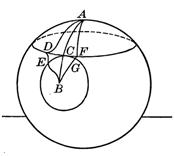微积分证明 下面我们利用球面坐标系与微积分给出一个精确的证明。令A,B是半径为R的球面上的任意两点，C为球心，大圆弧长可以表达为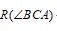以C为中心建立直角坐标系，让A在z轴上，则球面上任意一点P的坐标可以写成：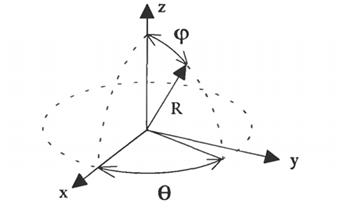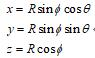空间中任意曲线的长度可以定义为：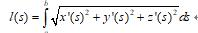其中s是参数，对球面曲线就有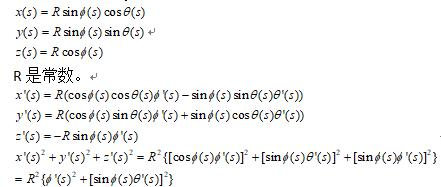所以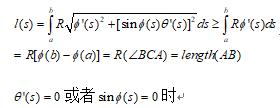上式严格成立，也就是要求不论s取值如何都不能离开大圆弧AB时等式严格成立，这就证明了球面上两点的最短距离为大圆弧。这个距离被高斯称为球面测地线。
展开全文• 判断几种特殊情况，点为多边形顶点，点在多边形边上，射线与多边形交点是多边形的顶点（多边形的每一个顶点其实是相邻两条线段的端点，所以顶点可以算两个点） /** * 给定点和多边形，判断给定的点是否在多边形...

坐标是否在指定区域坐标内
数学模型中，射线法求取射线与多边形交点个数来判断点是否在多边形内，交点为奇数时在多边形内，偶数在多边形外
判断几种特殊情况，点为多边形上顶点，点在多边形边上，射线与多边形交点是多边形的顶点（多边形的每一个顶点其实是相邻两条线段的端点，所以顶点可以算两个点）

/**
* 给定点和多边形，判断给定的点是否在多边形内
* @param point
* @param points
* @return
*/
public static boolean IsPtInPoly(Point2D.Double point, List<Point2D.Double> points){
int N = points.size();
boolean boundOrVertex = true; //如果点位于多边形的顶点或边上，也算做点在多边形内，直接返回true
int intersectCount = 0;//cross points count of x
double precision = 2e-10; //浮点类型计算时候与0比较时候的容差
Point2D.Double p1, p2;//neighbour bound vertices
Point2D.Double p = point; //当前点

p1 = points.get(0);//left vertex
for(int i = 1; i <= N; ++i){//check all rays
if(p.equals(p1)){
return boundOrVertex;//p is an vertex
}

p2 = points.get(i % N);//right vertex
if(p.x < Math.min(p1.x, p2.x) || p.x > Math.max(p1.x, p2.x)){//ray is outside of our interests
p1 = p2;
continue;//next ray left point
}

if(p.x > Math.min(p1.x, p2.x) && p.x < Math.max(p1.x, p2.x)){//ray is crossing over by the algorithm (common part of)
if(p.y <= Math.max(p1.y, p2.y)){//x is before of ray
if(p1.x == p2.x && p.y >= Math.min(p1.y, p2.y)){//overlies on a horizontal ray
return boundOrVertex;
}

if(p1.y == p2.y){//ray is vertical
if(p1.y == p.y){//overlies on a vertical ray
return boundOrVertex;
}else{//before ray
++intersectCount;
}
}else{//cross point on the left side
double xinters = (p.x - p1.x) * (p2.y - p1.y) / (p2.x - p1.x) + p1.y;//cross point of y
if(Math.abs(p.y - xinters) < precision){//overlies on a ray
return boundOrVertex;
}

if(p.y < xinters){//before ray
++intersectCount;
}
}
}
}else{//special case when ray is crossing through the vertex
if(p.x == p2.x && p.y <= p2.y){//p crossing over p2
Point2D.Double p3 = points.get((i+1) % N); //next vertex
if(p.x >= Math.min(p1.x, p3.x) && p.x <= Math.max(p1.x, p3.x)){//p.x lies between p1.x & p3.x
++intersectCount;
}else{
intersectCount += 2;
}
}
}
p1 = p2;//next ray left point
}

if(intersectCount % 2 == 0){//偶数在多边形外
return false;
} else { //奇数在多边形内
return true;
}
}

区域外点计算距离区域的最短距离
获取距离最短的顶点，取以这个顶点为端点的相邻两条线段，计算目标坐标点到两条线段的距离，较小值就为最短距离
海伦公式，知道三边长得到三角形面积
先判断三角形是否以中间点为顶点的钝角三角形，是的话返回相应点到点距离
然后用海伦公式算出高，返回高

/**
* luming
* 点到边的最短距离
* @param pointP
* @param pointA
* @param pointB
* @return
*/
public static double minDistanceToLine(Location pointP, Location pointA, Location pointB){

double pa = getDistance(pointP,pointA);
double pb = getDistance(pointP,pointB);
double ab = getDistance(pointA,pointB);

if((Math.pow(pa,2) + Math.pow(ab,2)) <= Math.pow(pb,2) ){
return pa;
}
if((Math.pow(pb,2) + Math.pow(ab,2)) <= Math.pow(pa,2) ){
return pb;
}

double p = (pa + pb + ab) / 2 ;
double s = Math.sqrt(p*(p-pa)*(p-pb)*(p-ab));

return (2*s/ab);
}

/**
* 通过经纬度获取距离(单位：米)
* @param lat1
* @param lng1
* @param lat2
* @param lng2
* @return
*/
public static double getDistance(double lat1, double lng1, double lat2,
double lng2) {
double s = 2 * Math.asin(Math.sqrt(Math.pow(Math.sin(a / 2), 2)
* Math.pow(Math.sin(b / 2), 2)));
s = Math.round(s * 10000d) / 10000d;
s = s*1000;
return s;
}


展开全文• 在一个 2 x 3 的板（board）有 5 块砖瓦，用数字 1~5 来表示, 以及一块空缺用 0 来表示. 一次移动定义为选择 0 与一个相邻的数字（上下左右）进行交换. 最终当板 board 的结果是 [[1,2,3],[4,5,0]] 谜板被解开。 ...BFS
• 为何地图上的航线是曲线如果我们观察地图上的航线，就会发现航线是弯曲的。基本上可以认为地球是个球体，如果飞机在两个城市之间飞行，最好的飞行线路是取这两个城市之间的最短距离。这其实课看成球面上任意两点之间...
• * 表面同一经线圈相差1"两点间的距离为 2πR/360/3600 * 表面同一纬线圈相差1"两点间的距离为 2πR×cos(纬度)/360/3600 * 当R取半径平均值6371km时， * 地球表面同一经线圈相差1"两点间的距离约为...
• 给你一个地图，类似于迷宫一样，中间有些障碍物，再给定起点终点，求该两点间最短路，显然，上述两种算法就不适用了，因为提到的迷宫，我们可能会很容易想到广搜bfs，但一次bfs下来实际求出了起点到所有点的最短...算法 A-Star dijkstra
• 我们在地图上看航线的时候，特别是长距离跨国航线，发现在地图上的航线并不是两点间的直线，而是弯曲的弧线。...而地球是球形的，很多人没有意识到在球体中，平面上这段距离并不是这两点间的最短距离。我们通过Goog...
• 虽然这头神奇的牛不能跳到树和石头，但是它可以在牧场随意跳，我们把牧场用一个x，y的坐标图来表示。 这头神奇的牛像其它牛一样喜欢吃草，给你一张地图，上面标注了The Knight的开始位置，树、灌木、石头以及...
• 题目保证对任意的查询请求，地图上都至少存在一条可达路线。 输入格式： 输入在第一行给出两个正整数N（2≤N≤500）N（2 \leq N \leq 500）N（2≤N≤500）和MMM，分别为地图中所有标记地点的个数和连接...
• 这些点都是中国地图上的一些点，并且是这些点的经纬度都是已经知道的，大约一共有139个城市c语言
• hihocoder-1931-最短管道距离 ...在一张2D地图上有N座城市，坐标依次是(X1, Y1), (X2, Y2), ... (XN, YN)。 现在H国要修建一条平行于X轴的天然气主管道。这条管道非常长，可以认为是一条平行于X轴的直线...
• 在一张2D地图上有N座城市，坐标依次是(X1, Y1), (X2, Y2), ... (XN, YN)。 现在H国要修建一条平行于X轴的天然气主管道。这条管道非常长，可以认为是一条平行于X轴的直线。 小Ho想知道如何修建这条管道，可以使N...
• 在一张2D地图上有N座城市，坐标依次是(X1, Y1), (X2, Y2), ... (XN, YN)。 现在H国要修建一条平行于X轴的天然气主管道。这条管道非常长，可以认为是一条平行于X轴的直线。 小Ho想知道如何修建这条管道，可以使N...算法 c++
• 在一张2D地图上有N座城市，坐标依次是(X1, Y1), (X2, Y2), … (XN, YN)。 现在H国要修建K条平行于X轴的天然气主管道。这些管道非常长，可以认为是一条条平行于X轴的直线。 小Ho想知道如何修建这K条管道，可以使N座...
• 你现在手里有一份大小为 N x N 的『地图』（网格） grid，上面的每个『区域』（单元格）都用 0 和 1 标记好了。其中 0 代表海洋，1 代表陆地，你知道距离陆地...如果我们的地图上只有陆地或者海洋，请返回动态规划
• 假如你有一张地图，地图上给出了每一对相邻城市的距离，从一个地点到另外一个地点，如何找到一条最短的路？ 最短路算法要解决的就是这类问题。定义：给定一个有(无)向图，每一条边有一个权值 w，给定一个起始点 S 和...
• 在一张2D地图上有N座城市，坐标依次是(X1, Y1), (X2, Y2), ... (XN, YN)。 现在H国要修建一条平行于X轴的天然气主管道。这条管道非常长，可以认为是一条平行于X轴的直线。 小Ho想知道如何修建这条管道，可以使N...
• 假如你有一张地图，地图上给出了每一对相邻城市的距离，从一个地点到另一个地点，如何找到一条最短的路？最短路算法要解决的就是这类问题。定义：给定一个有（无）向图，每一条边有一个权值w，给定一个起始点S和终止...
• 问题背景：地图上有很多个城市，已知各城市之间距离（或者是所需时间，后面都用距离了），一般问题无外乎就是以下几个： 从某城市到其余所有城市的最短距离【单源最短路径】 所有城市之间相互的最短距离【任意两...
• 最短路径问题下图给出了一个地图地图中每个顶点代表一个城市，两个城市间的连线代表道路，连线的数值代表道路长度。现在，我们想从城市a到达城市E。怎样走才能使得路径最短最短路径的长度是多少？设DiS［x］为...
• 题目保证对任意的查询请求，地图上都至少存在一条可达路线。 输入格式： 输入在第一行给出两个正整数N（2 ≤ N ≤ 500）和M，分别为地图中所有标记地点的个数和连接地点的道路条数。随后M行，每行按如下格式给出一条...算法 dijkstra 队列
• 题目保证对任意的查询请求，地图上都至少存在一条可达路线。 输入格式： 输入在第一行给出两个正整数N（2 ）和M，分别为地图中所有标记地点的个数和连接地点的道路条数。随后M行，每行按如下格式给出一条道路的...
• 　最短路径算法，顾名思义就是求解某点到某点的最短距离、消耗、费用等等，有各种各样的描述，在地图上看，可以说是图上一个地点到达另外一个地点的最短距离。比方说，我们把地图上的每一个城市想象成一个点，从...
• 一、前言　最短路径算法，顾名思义就是求解某点到某点的最短距离、消耗、费用等等，有各种各样的描述，在地图上看，可以说是图上一个地点到达另外一个地点的最短距离。比方说，我们把地图上的每一个城市想象成一...dijkstra 算法
• ]写一点我对最短路径算法的理解最短路径算法的作用就是在图中找出任意两点间最短距离的途径，比如可以在地图上找出任两个城市之间路程最短的那条路径。具体运用请见：...算法...